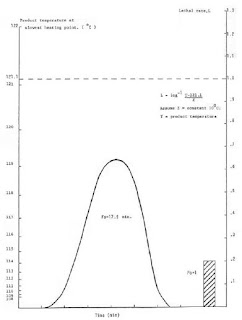# Calculating Fo Values in Fishery Products Canning: The improved general method

A plot of temperature versus time is made on specially constructed lethal rate paper which has on its left-hand vertical axis product temperature (on a log scale) while on the other vertical axis is drawn lethal rate (on a linear scale). Thus for each temperature can be shown the corresponding lethal rate. Time is plotted along the horizontal axis, using a convenient scale.

The area under the graph which represents the product of exposure time at all lethal rates throughout the process, is then divided by the area equivalent to that of an Fo value of unity. This yields the total sterilising effect, or the Fo value, for the process. In Figure 3 is shown a hypothetical heat penetration curve for a semi-solid product processed for 40 min at 120 ºC.Figure 3. Heat penetration lethal rate curve

The temperature profile shown is that of the slowest heating point. By counting squares or using a planimeter the area under the graph is found to be 71 cm², while the area corresponding to one unit of lethality (Fo = 1) is 4 cm². Therefore the total process lethality can be calculated,

This means the total sterilising effect of the process is equivalent to 17.5 minutes at 121.1 ºC, assuming instantaneous heating and cooling. We have now expressed the severity of sterilisation, as experienced at the slowest heating point of the can.

In the worked example, the retort was not operating at the reference temperature (121.1 ºC) nor did the product reach retort temperature. It is important not to confuse the specification for the process (40 min/120 ºC) with Fo for the process. A process specification alone indicates little about the total process lethality. It would be possible to have a process specification of 60 min at 121.1 ºC and Fo values of, say, 6.2 min and 11.5 min for 450-g and 225-g cans respectively, the different process severity in this case reflecting can size. Similar mode of heating (convection/conduction), pack weight and fill temperature can all affect the Fo value even though retorting conditions may be constant.

To Summarize:
• The improved general method takes account of the entire heating and cooling effects including any changes in heat penetration rates caused by product gelation or liquefaction.
• The thermal characteristics of the product need not be known. While this makes Fo calculation simple it limits the versatility of the technique .
• Theoretically the thermal centre of a conduction heating can is at the geometric centre, while that for a convection heating product is slightly below this on the vertical axis. It is important to locate thermocouple tips at the thermal centre for solid packs heating by conduction but less so for convection heating, as the rapid heat transfer caused by convection currents prevents any significant heating lags.

PAGE    1   -   2   -   3   -   4   -   5   -    -   7